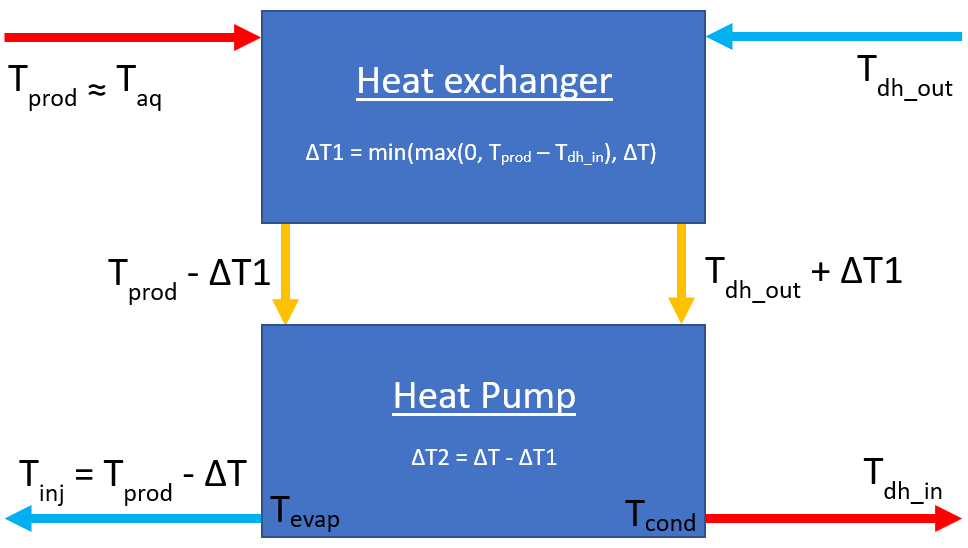# Heat pumps

The heat pump option in ThermoGIS allows for a lower injection temperature than specified by the return temperature of the district heat network (i.e. the inlet temperature of the heat exchanger / heat pump Tdh_in. It is assumed that the injection temperature Tinj is chosen as low as possible to maximize geothermal power, however limited to a maximum offset:

Tinj = Tres - ΔT

with:

• Tinj = injection temperature
• Taq = aquifer temperature
• ΔT = temperature difference between produced and injected water

ΔT is set to 40°C in accordance with recommendations from SodM. In addition, Tinj is set to a minimum of 5°C.

In the current online version of ThermoGIS the COP is set to a constant value of 5, and the lowered cooling temperature to 20°C (additional ΔT = 10). In a new implementation for district heating (DH) networks which is not yet available online, the COP of the heat pump is calculated based on the variable heat network inlet temperature (i.e. the outlet temperature of the heat exchanger / heat pump which is the inlet temperature of the district heating network Tdh_in and the injection temperature of the brine). The COP of the heat pump is given by the following equation:

COPhp = ηrel (Tdh_out + 273.1 + 3) / (Tdh_out - Tinj + 6)

with:

• COPhp = coefficient of performance of the heat pump
• ηrel = relative efficiency of the heat pump (0.6)
• Tdh_out = inlet temperature of the heat network / exit temperature of the heat pumpThe figure above shows a schematic representation of the system. On the left is the primary circuit of geothermal brine with temperature Tprod, assumed equal to the aquifer temperature Taq. The temperature is first lowered by amount ΔT1 in the heat exchanger. Next, the temperature is lowered by amount ΔT2 in the heat pump to the injection temperature Tinj. On the right hand side is the district heating network. The cool water returning from the network at temperature Tdh_out is first heated in the heat exchanger to Tdh_out + ΔT1, and then by the heatpump to the heat network inlet temperature Tdh_in. It is assumed that condenser and evaporator temperatures of the heatpump are respectively 3°C lower and higher than the source and outlet temperature of the heat pump.

The power reported in ThermoGIS corresponds to the 'pure' geothermal power, which in turn corresponds to the product of flow rate, ΔT, and volumetric heat capacity of the brine. The COP reported in ThermoGIS corresponds to the ratio of the produced final thermal power (which is equal to the geothermal power, topped up by the electricity consumption in the heat pump) and the sum of power consumption in the heat pump and ESP. Please note that the COPhp accounts for the heat produced in the heat pump only. If Taq > Tdh_in then the heating is partly provided by the heat exchanger, and the remaining part by the heat pump. Therefore the COP in the heat pump scenario can be significantly higher than COPhp.